367
Disjoint Set Union (Union Find)
Code Monk
Disjoint-sets
Union Find

The efficiency of an algorithm sometimes depends on using an efficient data structure. A good choice of data structure can reduce the execution time of an algorithm and Union-Find is a data structure that falls in that category.

Let’s say, you have a set of N elements which are partitioned into further subsets, and you have to keep track of connectivity of each element in a particular subset or connectivity of subsets with each other. To do this operation efficiently, you can use Union-Find Data Structure.

Let’s say there are 5 people A, B, C, D E. A is a friend of B, B is a friend of C and D is a friend of E. As we can see:

1) A, B and C are connected to each other.
2) D and E are connected to each other.

So we can use Union Find Data Structure to check whether one friend is connected to another in a direct or indirect way or not. We can also determine the two different disconnected subsets. Here 2 different subsets are {A, B, C} and {D, E}.

You have to perform two operations here :

Union (A, B) - connect two elements A and B. Find (A, B) - find, is there any path connecting two elements A and B

Example: You have a set of elements S = {0, 1, 2, 3, 4, 5, 6, 7, 8, 9}. Here you have 10 elements (N = 10 ).We can use an array Arr to manage the connectivity of elements. Arr[ ] indexed by elements of set, having size of N (as N elements in set) and can be used to manage the above operations.

Assumption: A and B objects are connected only if Arr[ A ] = Arr [ B ].

Now how we will implement above operations :

Find (A, B) - check if Arr[ A ] is equal to Arr[ B ] or not. Union (A, B) - Connect A to B and merge the components having A and B by changing all the elements ,whose value is equal to Arr[ A ], to Arr[ B ].

Initially there are 10 subsets and each subset has single element in it.When each subset contains only single element, the array Arr is: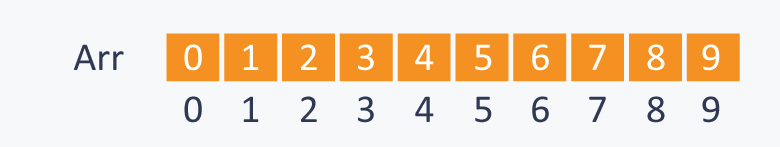Let’s perform some Operations: 1) Union(2, 1)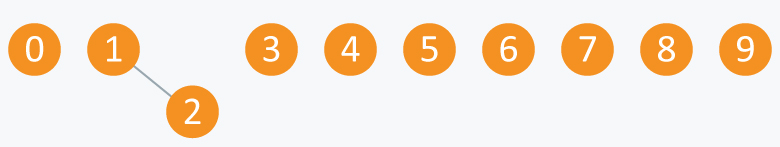Arr will be: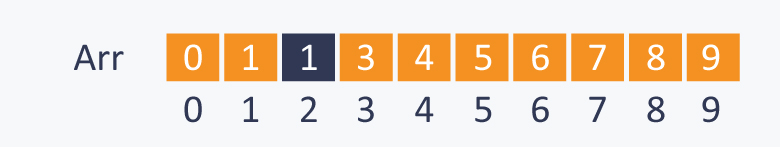2) Union(4, 3)
3) Union(8, 4)
4) Union(9, 3)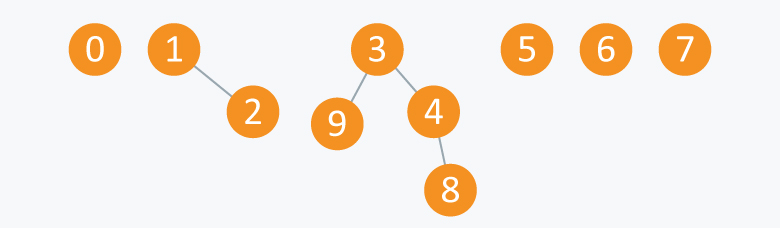Arr will be: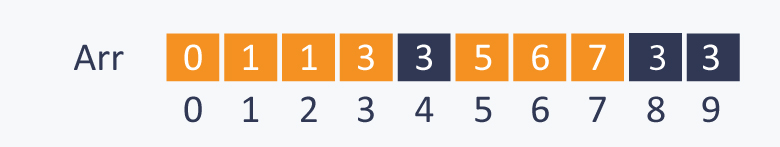5) Union(6, 5)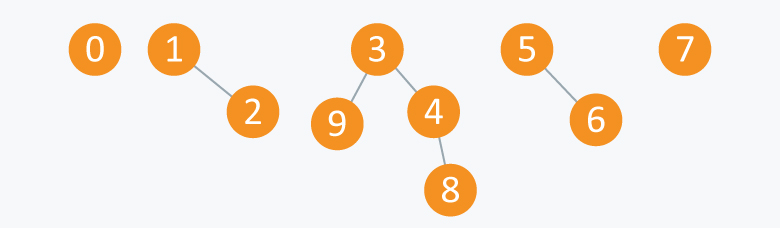Arr will be: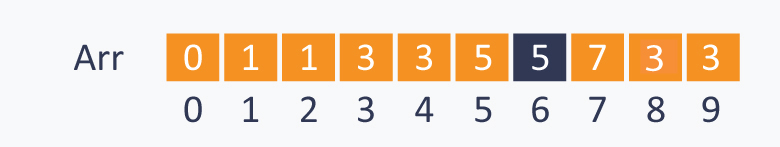After performing some operations of Union(A ,B), you can see that now there are 5 subsets. First has elements {3, 4, 8, 9}, second has {1, 2}, third has {5, 6}, fourth has {0} and fifth has {7}. All these subsets are said to be Connected Components.

One can also relate these elements with nodes of a graph. The elements in one subset can be considered as the nodes of the graph which are connected to each other directly or indirectly, therefore each subset can be considered as connected component.

From this, we can infer that Union-Find data structure is useful in Graphs for performing various operations like connecting nodes, finding connected components etc.

Let’s perform some Find(A, B) operations. 1) Find (0, 7) - as 0 and 7 are disconnected ,this will gives false result.
2) Find (8, 9) -though 8 and 9 are not connected directly ,but there exist a path connecting 8 and 9, so it will give us true result.

When we see above operations in terms of components, then :

Union(A, B) - Replace components containing two objects A and B with their union.
Find(A, B) - check if two objects A and B are in same component or not.

So if we perform operation Union(5, 2) on above components, then it will be :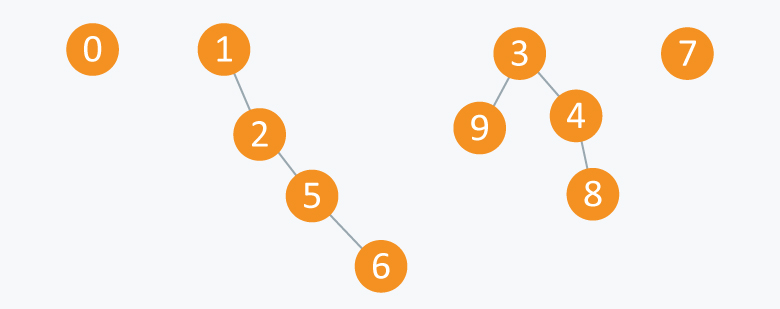Now the Arr will be: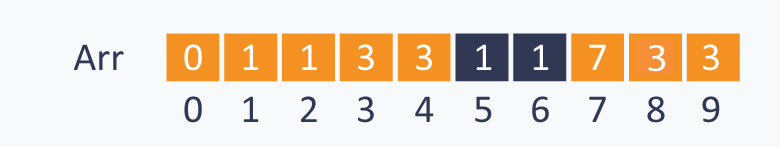Implementation:

Initially there are N subsets containing single element in each subset, so to initialize array we will use initialize ( ) function.

void initialize( int Arr[ ], int N)
{
for(int i = 0;i<N;i++)
Arr[ i ] = i ;
}
//returns true,if A and B are connected, else it will return false.
bool find( int Arr[ ], int A, int B)
{
if(Arr[ A ] == Arr[ B ])
return true;
else
return false;
}
//change all entries from Arr[ A ] to Arr[ B ].
void union(int Arr[ ], int N, int A, int B)
{
int TEMP = Arr[ A ];
for(int i = 0; i < N;i++)
{
if(Arr[ i ] == TEMP)
Arr[ i ] = Arr[ B ];
}
}


As loop in Union function iterates through all the N elements for connecting two elements. So performing this operation on N objects will take O(N2) time, which is quite inefficient.

Let’s try another approach:

Idea: Arr[ A ] is a parent of A.

We can consider a root element of each subset, which is a only special element in that subset having itself as the parent. Let’s say R is a root element, then Arr[ R ] = R.

To make it more clear,let’s take a subset S = {0, 1, 2, 3, 4, 5}

Initially each element is the root of itself in all subsets, as Arr[ i ] = i, where i is element in the set, therefore root(i) = i.Performing Union(1, 0) will connect 1 to 0 and will set root(0) as the parent of root(1). As root(1) = 1, and root(0) = 0, therefore value of Arr[ 1 ] will be changed from 1 to 0. It will make 0 as a root of subset containing elements {0, 1}.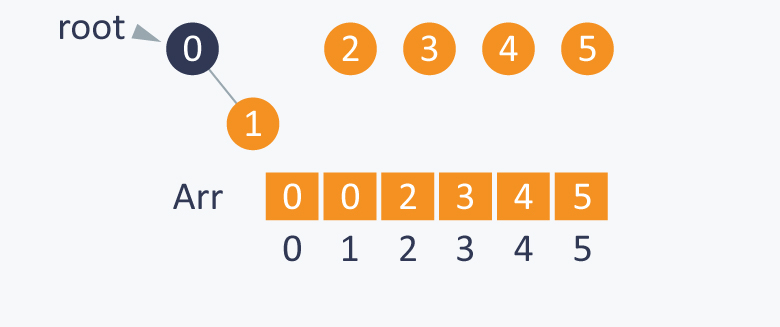Now performing Union(0, 2), will indirectly connect 0 to 2, by setting root(2) as the parent of root(0). As root(0) is 0 and root(2) is 2, therefore it will change value Arr[ 0 ] from 0 to 2. Now 2 will be the root of subset containing elements {2, 0, 1}.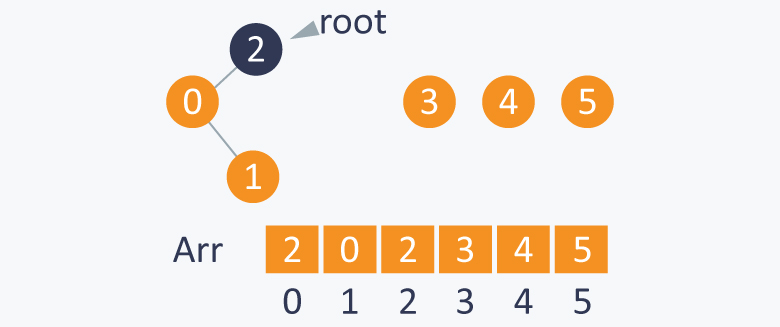Similarly Union(3, 4) will indirectly connect 3 to 4, by setting root(4) as the parent of root(3). As root(3) is 3 and root(4) is 4, therefore it will change value of Arr[ 3 ] from 3 to 4. It will make 4 as a root of subset containing elements {3, 4}.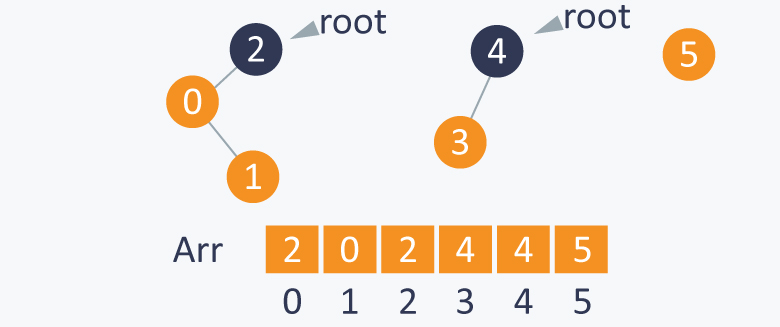Performing Union(1, 4 ) will indirectly connect 1 to 4, by setting root(4) as the parent of root(1). As root(4) is 4 and root(1) is 2, therefore it will change value of Arr[ 2 ] from 2 to 4. It makes 4 as root of set containing elements {0, 1, 2, 3, 4}.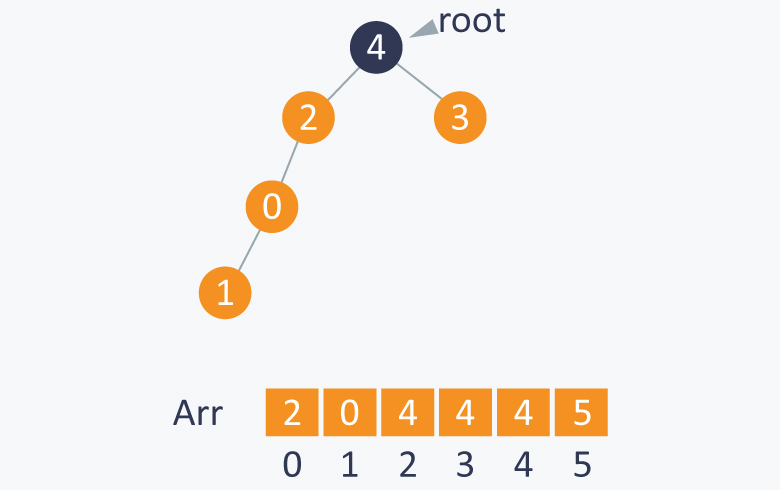After each step you can observe the change in array Arr also.
After performing required Union(A, B) operations, we can easily perform the Find(A, B) operation to check whether A and B are connected or not. It can be checked by calculating roots of both A and B. If roots of A and B are same, that means both A and B are in same subset and are connected.

Now how to calculate root of a element ?
As we know that Arr[ i ] is the parent of i (where i is the element of set),then the root of i is Arr[ Arr[ Arr[ …...Arr[ i ]...... ] ] ] until Arr[ i ] is not equal to i. Simply we can run a loop until we get a element which is a parent of itself.

Note: This can be only done when there is no cycle in the elements of subset, otherwise loop will run infinitely.

Find(1, 4) - 1 and 4 have same root as 4, therefore it means they are connected and this operation will give true as a result.

Find(3, 5) - 3 and 5 do not have same root, as root(3) is 4 and root(5) is 5. It means they are not connected and it will give false as a result.

Implementation:

As initially all the elements are parent of itself,which can be done using initialize function discussed above.

//finding root of an element.
int root(int Arr[ ],int i)
{
while(Arr[ i ] != i)           //chase parent of current element until it reaches root.
{
i = Arr[ i ];
}
return i;
}

/*modified union function where we connect the elements by changing the root of one of the element */

int union(int Arr[ ] ,int A ,int B)
{
int root_A = root(Arr, A);
int root_B = root(Arr, B);
Arr[ root_A ] = root_B ;       //setting parent of root(A) as root(B).
}
bool find(int A,int B)
{
if( root(A)==root(B) )       //if A and B have same root,means they are connected.
return true;
else
return false;
}


Now as you can see, in worst case, this idea will also take linear time in connecting 2 elements and even in finding that if two elements are connected or not, it will take linear time.
Another disadvantage is that while connecting two elements, we do not check which subset has more element than other and sometimes it creates a big problem as in worst case we have to perform approximately linear time operations.

We can avoid this, by keeping the track of size of each subset and then while connecting two elements, we can connect the root of subset having smaller number of elements to the root of subset having larger number of elements.

Example:

Here if we want to connect 1 and 5, then we will connect the root of Subset A (subset which contains 1) will be connected to root of Subset B (contains 5), this is because Subset A contains less number of elements than of Subset B.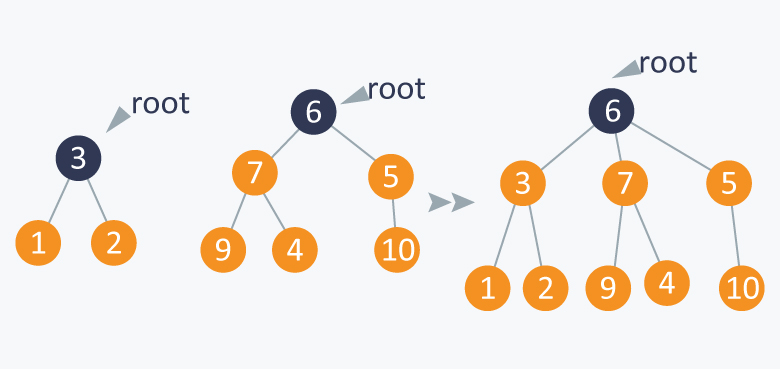It will balance the tree formed by the above operations. We call this operation as weighted_union operation .

Implementation:

Initially the size of each subset will be one as each subset will have only one element and we can initialize it in the initialize function discussed above:

size[ ] array will keep track of size of each subset.

//modified initialize function:
void initialize( int Arr[ ], int N)
{
for(int i = 0;i<N;i++)
{
Arr[ i ] = i ;
size[ i ] = 1;
}
}


root() and find() function will be same as above .

Union function will be modified as we will connect two subsets according to the number of elements in subset.

//modified union function

void weighted-union(int Arr[ ],int size[ ],int A,int B)
{
int root_A = root(A);
int root_B = root(B);
if(size[root_A] < size[root_B ])
{
Arr[ root_A ] = Arr[root_B];
size[root_B] += size[root_A];
}
else
{
Arr[ root_B ] = Arr[root_A];
size[root_A] += size[root_B];
}

}


Example:

You have a set S = {0, 1, 2, 3, 4, 5} Initially all the subsets have a single element and each element is a root of itself. Initially size[ ] array will be :Perform Union(0, 1). Here we can connect any root of any element with root of other one as both the element’s subsets have same size and then we will update the respective size.
If we connect 1 to 0 and make 0 as a root and then size of 0 will change from 1 to 2.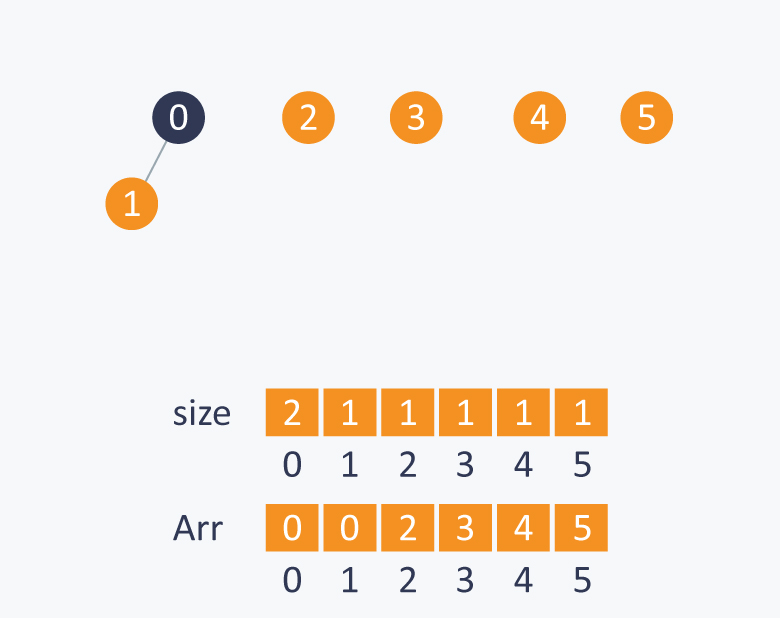While performing Union(1, 2), we will connect root(2) with root(1) as subset of 2 has less number of elements than number of elements in subset of 1.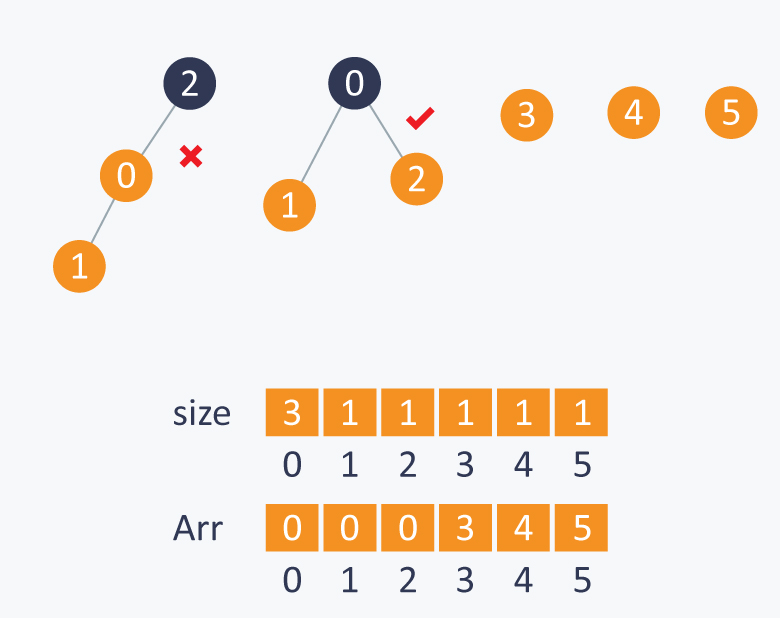Similarly in Union(3, 2), it will connect root(3) to root(2) as subset of 3 has less number of element than number of elements in subset of 2.Maintaining a balance tree, will reduce complexity of union and find function from N to log2N.

Can we improve more ?

Idea: Union with path compression : While computing the root of A, set each i to point to its grandparent (thereby halving the path length), where i is the node which comes in between path, while computing root of A.

// modified root function.

int root (int Arr[ ] ,int i)
{
while(Arr[ i ] != i)
{
Arr[ i ] = Arr[ Arr[ i ] ] ;
i = Arr[ i ];
}
return i;
}


When we use Weighted-union with path compression it takes log * N for each union find operation,where N is the number of elements in the set.

log *N is the iterative function which computes the number of times you have to take log of N till the value of N doesn’t reaches to 1.

log*N is much better than log N, as its value reaches at most up to 5 in the real world.

Applications:

1) As explained above, Union-Find is used to determine the connected components in a graph. We can determine whether 2 nodes are in the same connected component or not in the graph. We can also determine that by adding an edge between 2 nodes whether it leads to cycle in the graph or not.
We learned that we can reduce its complexity to a very optimum level, so in case of very large and dense graph, we can use this data structure.

2) It is used to determine the cycles in the graph. In the Kruskal’s Algorithm, Union Find Data Structure is used as a subroutine to find the cycles in the graph, which helps in finding the minimum spanning tree.(Spanning tree is a subgraph in a graph which connects all the vertices and spanning tree with minimum sum of weights of all edges in it is called minimum spanning tree).

Practice Problems:
1) Panda and Combination